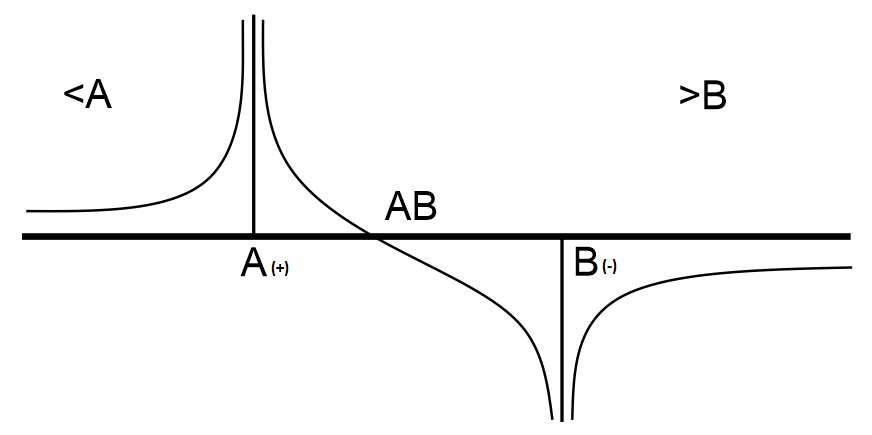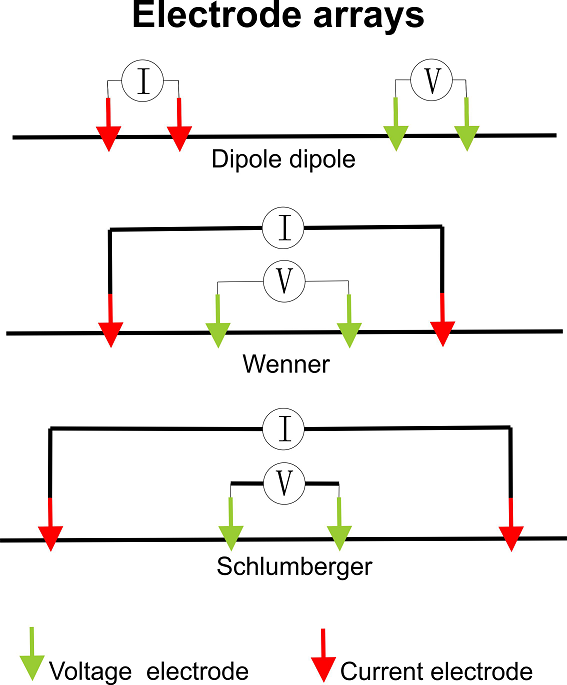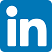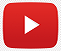NEWS CENTER

# ZZ Array NEW CONCEPT

## Release time：2020-08-20

ZZ-Array:

Designed For Full-Channel Systems and Inversion

ZZ-Array and Full-Channel technology are created by ZZ Resistivity Imaging Pty Ltd, Australia

# Basic principle of the resistivity method

For the resistivity method, firstly we need two electrodes (A and B) to inject current into the ground to form an electric field. When two electrodes (Figure 1) representing current electrodes at source A and sink B, inject current into the ground which has a uniform resistivity; an electric field will be formed in the half space. If multiple electrodes are placed on the surface to measure the ground’s electric potential, then a voltage curve similar to the curve in Figure 2 will be obtained.Figure 1. Calculated electric field distribution images for a uniformly resistive background.Figure 2. Voltage (potential) curve produced by a surface resistivity survey through a uniform medium, marking three sections: The left side of A, between A and B (AB), and the right side of B.

The medium below the surface is often not uniform, instead it can be anomalistic. For example, it may contain a high resistivity (Figure 3), thus the potential curve on the surface is no longer the same ideal form to that shown in Figure 2.  This is because the electrical field is distorted by this high resistivity . We can see this by comparing the two contours in Figure 1 and Figure 3, demonstrating that the potentials we collect on the surface contain the electric field distortion information. Therefore, we can use the collected potentials to calculate and find the underground target.  This is the basic principle of the resistivity method.Figure 3. Electric field calculated from the same uniform background but with a relatively low resistivity anomaly in the middle.

# The difference between multi-channel and multi-electrode

Currently, most resistivity instruments are multi-electrode systems, meaning there are more than 4 electrodes connected to the instrument during a survey; However, they may not be multi-channel resistivity systems.  The following diagram explains the difference between single channel, multi-channel and full-channel systems.Figure 4. Single-channel, mutli-channel and Full-Channel data collection comparison.

# Full-channel resistivity data collection

By comparing the two contours in Figure 1 and Figure 3, we can see that all potentials collected on the surface can be split into three sections: The left side of A, between A and B and the right side of B - each are distorted by the anomaly.  This means that all potentials collected from the three sections contain the distorted potential and provide useful data for later calculations.  Therefore, we should collect this information when possible.

Traditional resistivity data collection arrays such as Wenner, Schlumberger, dipole-dipole, gradient arrays, etc. (Figure 4), collect data from only one of the three sections comprising the potential surface curve (Figure 2).  For example, Wenner and Schlumberger collect data from the middle section between A and B, and the dipole-dipole array collects data from the right side of B.  The full-channel method will collect all potentials from all three sections (the left side of A, AB, and the right side of B) simultaneously.  Therefore, the full-channel method will collect much more potential information than any other arrays for each AB current injection.Figure 5. Illustration of commonly used traditional data collection arrays.

# ZZ-Array design

The ZZ-Array uses full-channel methodology, meaning it will use all electrodes (except A and B) to collect potential data from all three sections simultaneously.  For symmetry, it uses a similar principle to VES.

Supposing there are 64 electrodes in a survey, we set 60 VES central points. For each VES central point, we set N AB current injections (A and B in each pair are symmetric for the VES central point, at i*3-1, i=1 to N).  For example, for the central point at electrode 20, the first 3 AB current injection pairs are at (18,22), (15,25), and (12,28).  These 3 AB pairs are minimum for each central point in the ZZ-Array.  Furthermore, you can have the 4th AB current injection at (9,31) and the 5th at (6,34) for this central point.  A greater number of AB current injections for each central point will increase the exploration depth.

For one system with 64 electrodes, if we set 3 AB pairs for each central point, we will have a total of 162 AB current pairs * 61 voltages = 9,882 ABMN data for a surface survey using the ZZ-Array.  For 64 electrodes, we can have a maximum 330 AB current pairs with the ZZ-Array and will collect 330 * 61 = 20,130 ABMN data. For further information, please download the ZZ Universal demo data acquisition program from www.zzgeo.com and try it.

# ZZ Array data example (N=4 and 64 electrodes)

 ZZ surface array (N=4) No A B Central point ZZ surface array 1 1 5 3 ZZ surface array 2 2 6 4 ZZ surface array 3 3 7 5 ZZ surface array 4 4 8 6 ... ... ZZ surface array 57 57 61 59 ZZ surface array 58 58 62 60 ZZ surface array 59 59 63 61 ZZ surface array 60 60 64 62 ZZ surface array 61 1 11 6 ZZ surface array 62 2 12 7 ZZ surface array 63 3 13 8 ZZ surface array 64 4 14 9 ZZ surface array 65 5 15 10 ... ... ZZ surface array 157 43 59 51 ZZ surface array 158 44 60 52 ZZ surface array 159 45 61 53 ZZ surface array 160 46 62 54 ZZ surface array 161 47 63 55 ZZ surface array 162 48 64 56 ZZ surface array 163 1 23 12 ZZ surface array 164 2 24 13 ZZ surface array 165 3 25 14 ... ... ZZ surface array 201 39 61 50 ZZ surface array 202 40 62 51 ZZ surface array 203 41 63 52 ZZ surface array 204 42 64 53

Figure 7. example of ZZ array AB list with N=4 and 64 electrodes.

LAST：Full-Channel Resistivity/IP
NEXT： Cables: Customised For Any Instrument

ZZ Resistivity Imaging Pty. Ltd.

sales@zzgeo.comhttps://www.youtube.com/channel/UCIvYShsZNr0w_Nm3r3td06Q